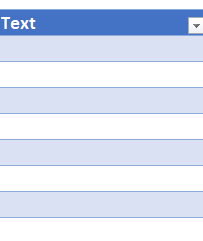In a workbook, I have two sheets.

• Sheet1 contains a table from Table Builder. The table is called Textmatrix.It contains one important column called Textcode. Textcode contains the same information like Nr. from Sheet2.
In this table I added a column called Text with the formular below:
`=@NP("Formula","=TEXTJOIN(CHAR(10),TRUE,IF([@Textcode]=INDIRECT(""Textblockrow[Nr.]"",1),INDIRECT(""Textblockrow[Text]"",1),""""))")`
• Sheet2 contains a table from Table Builder. The table is called Textblockrow and there a two columns important: Nr. and Text

However, the final result in column Text looks like this. It is empty. Because the formula looks like this:

`=TEXTJOIN(CHAR(10),TRUE,IF([@Textcode]=@INDIRECT("Textblockrow[Nr.]",1),INDIRECT("Textblockrow[Text]",1),""))`The reason is the @ sign in front of INDIRECT. If I remove it, the column gets refreshed and the formula works.

So how can I fix it?